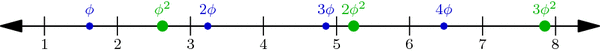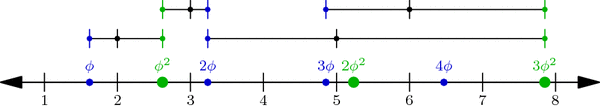# Just Beatty It

[This is the 6th post in the current series about Wythoff’s game: see posts #1, #2, #3, #4, and #5. Caveat lector: this post is a bit more difficult than usual. Let me know what you think in the comments!]

Our only remaining task from last week was to prove the mysterious Covering Theorem: we must show that there is exactly one dot in each row and column of the grid (we already covered the diagonal case). Since the rows and columns are symmetric, let’s focus on columns.

The columns really only care about the x-coordinates of the points, so let’s draw just these x-coordinates on the number-line. We’ve drawn $$\phi,2\phi,3\phi,\ldots$$ with small dots and $$\phi^2,2\phi^2,3\phi^2,\ldots$$ with large dots. We need to show that there’s exactly one dot between 1 and 2, precisely one dot between 2 and 3, just one between 3 and 4, and so on down the line. For terminology’s sake, break the number line into length-1 intervals [1,2], [2,3], [3,4], etc., so we must show that each interval has one and only one dot:Multiples of $$\phi$$ (small blue dots) and of $$\phi^2$$ (large green dots) perfectly interleave the integers on the number line. This is proved below.

Why is this true? One explanation hinges on a nice geometric observation: Take any small dot s and large dot t on our number-line above, and cut segment st into two parts in the ratio $$1:\phi$$ (with s on the shorter side). Then the point where we cut is always an integer! For example, the upper-left segment in the diagram below has endpoints at $$s=2\cdot\phi$$ and $$t=1\cdot\phi^2$$, and its cutting point is the integer 3:When a segment formed by a small and a large dot is cut into a $$1:\phi$$ ratio (closer to the small dot), the cutting point is always an integer. In fact, the segment between $$j\cdot \phi$$ and $$k\cdot \phi^2$$ is cut at the integer j+k.

In general, if s is the jth small dot—i.e., $$s=j\cdot\phi$$—and $$t=k\cdot\phi^2$$ is the kth large dot, then the cutting point between s and t is $$\frac{1}{\phi}\cdot s+\frac{1}{\phi^2}\cdot t = j+k$$ (Why?!). But more importantly, this observation shows that no interval has two or more dots: a small dot and a large dot can’t be in the same interval because they always have an integer between them!

So all we have to do now is prove that no interval is empty: for each integer n, some dot lies in the interval [n,n+1]. We will prove this by contradiction. What happens if no dot hits this interval? Then the sequence $$\phi,2\phi,3\phi,\ldots$$ jumps over the interval, i.e., for some j, the jth dot in the sequence is less than n but the (j+1)st is greater than n+1. Likewise, the sequence $$\phi^2,2\phi^2,3\phi^2,\ldots$$ jumps over the interval: its kth dot is less than n while its (k+1)st dot is greater than n+1:Illustrating the hypothetical situation where interval [n, n+1] contains no dot. This is used in a proof by contradiction to show that the interval in fact cannot be empty.

By our observation above on segment $$s=j\phi$$ and $$t=k\phi^2$$, we find that the integer j+k is less than n, so $$j+k\le n-1$$. Similarly, $$j+k+2 > n+1$$, so $$j+k+2 \ge n+2$$. But together these inequalities say that $$n\le j+k\le n-1$$, which is clearly absurd! This is the contradiction we were hoping for, so the interval [n,n+1] is in fact not empty. This completes our proof of the Covering Theorem and the Wythoff formula!

It was a long journey, but we’ve finally seen exactly why the Wythoff losing positions are arranged as they are. Thank you for following me through this!

### A Few Words on the Column Covering Theorem

Using the floor function $$\lfloor x\rfloor$$ that rounds x down to the nearest integer, we can restate the Column Covering Theorem in perhaps a more natural context. The sequence of integers $$\lfloor\phi\rfloor = 1, \lfloor 2\phi\rfloor = 3, \lfloor 3\phi\rfloor = 4, \lfloor 4\phi\rfloor = 6, \ldots$$ is called the Beatty sequence for the number $$\phi$$, and similarly, $$\lfloor\phi^2\rfloor = 2, \lfloor 2\phi^2\rfloor = 5, \lfloor 3\phi^2\rfloor = 7, \lfloor 4\phi^2\rfloor = 8,\ldots$$ is the Beatty sequence for $$\phi^2$$. Today we proved that these two sequence are complementary, i.e., together they contain each positive integer exactly once. We seemed to use very specific properties of the numbers $$\phi$$ and $$\phi^2$$, but in fact, a much more general theorem is true:

Beatty’s Theorem: If $$\alpha$$ and $$\beta$$ are any positive irrational numbers with $$\frac{1}{\alpha}+\frac{1}{\beta}=1$$, then their Beatty sequences $$\lfloor\alpha\rfloor, \lfloor 2\alpha\rfloor, \lfloor 3\alpha\rfloor,\ldots$$ and $$\lfloor\beta\rfloor, \lfloor 2\beta\rfloor, \lfloor 3\beta\rfloor,\ldots$$ are complementary sequences.

Furthermore, our same argument—using $$\alpha$$ and $$\beta$$ instead of $$\phi$$ and $$\phi^2$$—can be used to prove the more general Beatty’s Theorem!

### Notes

1. Hint: use the identity $$\frac{1}{\phi}+\frac{1}{\phi^2}=1$$. []
2. To be thorough, we should also check that no interval has two small or two large dots. Why can’t this happen? []

## 4 thoughts on “Just Beatty It”

1.Paul Salomon says:

This is one of the best series of math posts I’ve ever found online! GREAT WORK! I started my algebra 2 class with this game, which we called “Corner the Queen,” incidentally. Im really excited to do it again next year with all of this to back me up. Cheers!

1.Zachary Abel says:

Wow, thank you so much! I’m glad you enjoyed this series, and I hope you do find it useful in your teaching. Please let me know how it works out!

2.Brent Yorgey says:

Cool! I really enjoyed this series of posts.

Here’s a simpler proof that no interval is empty. Since $\phi < 2$, there can never be two consecutive intervals with no blue dots in them. That means that the two intervals on either side of the empty one must each contain a blue dot. But since we already know no two dots share the same interval, this makes a stretch of three consecutive intervals with no green dot — which is impossible since $\phi^2 < 3$. (To be sure, your proof does have the advantage of generalizing to Beatty's theorem, whereas mine is specific to $\phi$ and $\phi^2$.)

1.Zachary Abel says:

Thanks, Brent, and nice proof! That is certainly much simpler for $$\phi$$ and $$\phi^2$$. At the price of one extra step, I think we can extend it to the general case as well:

<proof>
Assume $$\alpha < 2 < \beta$$, and pick integer k with $$k < \beta < k+1$$, meaning $$1+\frac{1}{k} < \alpha < 1+\frac{1}{k-1}$$. Here's the extra step: if there were an empty interval I, then multiples of $$\alpha$$ would occupy at least k-1 consecutive intervals on either side of I. Indeed, the first multiple of $$\alpha$$ after I has fractional part less than $$\frac{1}{k-1}$$, the next has fractional part less than $$\frac{2}{k-1}$$, and so on k-1 times. The left side of I is similar. Now there are 2k-1 consecutive intervals with no multiple of $$\beta$$, so $$\beta > 2k-1$$. This contradicts $$\beta < k+1$$ because $$k\ge 2$$. </proof> Now that I think about it, this argument is almost identical to Solution 1 of Putnam 1995 B6 in the MAA's 1985–2000 Putnam Solutions and Commentary book.